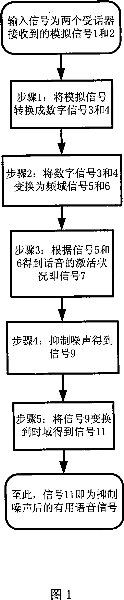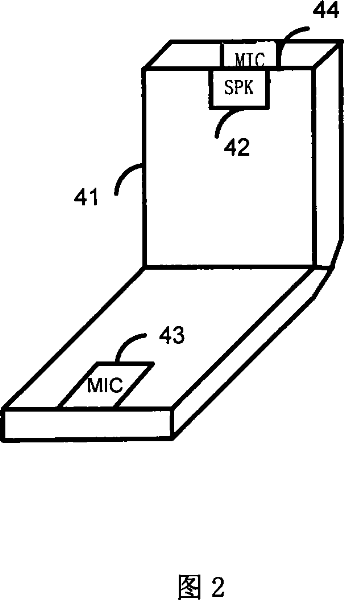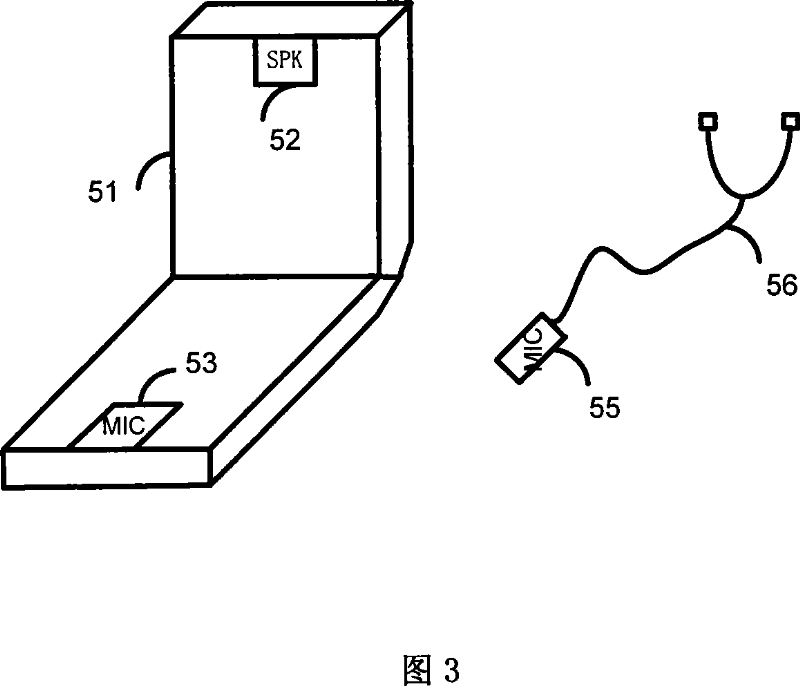# Method for suppressing voice noise of mobile phone

## A mobile phone and noise suppression technology, which is applied to the structure of the phone, voice analysis, and sidetone cancellation circuits, etc., can solve the problems of poor suppression effect, and achieve the effects of easy development and implementation, easy implementation, and simple structure

Inactive Publication Date: 2007-09-19

1 Cites 4 Cited by

## AI-Extracted Technical Summary

### Problems solved by technology

But in principle, its technical solution may not be effective in suppressing large-scale noise, such as radio broadcast...
View more

## Abstract

The present invention relates to a voice noise suppression method for a mobile phone, which pertains to the mobile phone technology. The method includes: establishing a receiver, wherein the distance between the receiver and a receiver of mobile telephone using the method makes the energy of the obtained voice in mobile phone receiver greater than that of the obtained voice in the receiver, and the noise energy obtained by the two receiver is in the same order of magnitude; respectively receiving analog signals of the two receivers, and converting analog signals into digital signals; converting the two output digital signals into frequency domain signals; detecting the voice activation state according to the frequency domain signals; inhibiting noise based on the voice activation state to obtain the noise-inhibiting frequency domain signals; and outputing useful voice signals after converting the frequency domain signals into time domain signals. The present invention not only has characteristics of a simple structure, easy to achieve, low-cost, significant inhibitory effect of the mobile phone voice noise, and facilitating the implementation and the development; but also could be applied to any occasion.

Application Domain

Speech analysisAnti-side-tone circuits +2

Technology Topic

Order of magnitudeFrequency domain +10

## Image

•••## Examples

• Experimental program(1)

### Example Embodiment

 A method for suppressing voice noise in a mobile phone proposed by the present invention is described in detail as follows with reference to the drawings and embodiments:
 The method of the present invention requires a receiver, and the distance between the receiver and the receiver of the mobile phone using the method is such that the energy of the voice obtained by the receiver of the mobile phone is greater than the energy of the voice obtained by the receiver, and the two receivers obtain The noise energy of is of the same order of magnitude; the overall flow of the method of the present invention is shown in Figure 1. The method includes the following steps:
 1) Receiving the analog signals 1 and 2 output by the two receivers respectively, and converting the analog signals into digital signals 3 and 4;
 2) Transform the two output digital signals 3 and 4 into frequency domain signals 5 and 6;
 3) Detect the voice activation status according to the frequency domain signal and output signal 7;
 4) The output signal 7 according to the voice activation status suppresses noise to obtain a noise suppressed frequency domain signal 9;
 5) After transforming the frequency domain signal 9 into a time domain signal 11, a useful voice signal is output.
 The two receivers of the present invention can be implemented in a variety of ways, for example:
 The first implementation is shown in FIG. 2, the first receiver is the original receiver 43 of the mobile phone 41, and 42 is the original normal speaker. The second receiver is a newly installed receiver 44 (the specific installation method is conventional technology), which can be located on the back of the speaker 42 of the mobile phone at a distance 43 and can also receive noise well.
 The two receivers of the present invention are not limited to the above two implementation modes, and any two receivers obtained by other methods according to the principle of the present invention also belong to the scope of the present invention.
 The working principle of using the method of the present invention to suppress the voice noise of the mobile phone is explained as follows:
 The module for suppressing voice noise made by the method of the present invention is installed in a suitable place in the phone case, and the two input ends of the module are respectively connected to the above two receivers, and the output ends are connected to the input ends of the telephone. (Ie the port where the original telephone receiver is connected) can work.
 In Figure 2 or Figure 3, use a and b to represent the distance between the two receivers and the speaker’s mouth, Ea and Eb to represent the energy of the useful voice signal received by the two receivers, and e and f to represent the noise The distance between the source and the two receivers, Ee and Ef are used to represent the energy of the noise received by the two receivers. Then the sound energy propagation attenuation is inversely proportional to the square of the distance. Under the reasonable assumptions b>>a (“>>” means much greater than) and f~e (“~” means approximately equal to), there is Ea /Eb>>1 and Ee/Ef~1. Signal 1 corresponds to the analog signal output by the receiver 43 in FIG. 2 or the receiver 55 in FIG. 3; signal 2 corresponds to the analog signal output by the receiver 44 in FIG. 2 or the receiver 53 in FIG. 3. The energy of signal 1 corresponds to Ea and Ee, and the energy of signal 2 corresponds to Eb and Ef. It can be known that signal 1 is composed of useful signals and noise signals, and signal 2 is composed of useful signals and noise signals of very small intensity. When the speaker speaks, signal 1 has a greater intensity than signal 2. When the speaker is not speaking, there is no useful signal, then signal 1 and signal 2 have basically the same strength.
 Signals 3 and 4 are digital signals obtained by analog-to-digital conversion of signals 1 and 2, respectively, and frequency-domain signals are obtained after frequency-domain conversion of the digital signals. Voice activation detection judges whether the speaker is speaking by calculating the spectrum intensity of 5 and 6, that is, voice activation detection. Signal 7 is used to indicate this voice activation. When voice is activated, noise is suppressed by spectral amplitude subtraction and removal of residual noise components. When voice is not activated, noise suppressed signal is obtained by spectral amplitude subtraction and removing more residual noise components. Then, the frequency domain signal 9 output after noise suppression is transformed into the time domain and superimposed as a useful signal 11 to be transmitted to the call partner.
 The embodiments of each step of the present invention are respectively described as follows:
 In step 1) of the method of the present invention, a conventional analog-to-digital converter can be used to convert the analog signals output by the two receivers into digital signals.
 In step 2) of the method of the present invention, the specific implementation method of transforming the two output digital signals into frequency domain signals is shown in Fig. 4, which performs serial-to-parallel conversion, windowing and Fourier transformation on the two input signals. The relationship between the output signal and the input signal and the specific calculation methods of serial-to-parallel conversion, windowing and Fourier transform are described in detail as follows:
In this embodiment, in order to facilitate processing, the two signals in the analog-to-digital conversion have the same sampling frequency. If they are different, the incoming signals 3 and 4 can have the same sampling frequency through up-sampling or down-sampling. It is also common to assume that signals 3 and 4 have the same sampling frequency. Let fs represent the sampling frequency. The frequency domain transform method is to process the input digital signals 3 and 4 in a frame manner. Use s3(n) and s4(n) to represent the values ​​of signals 3 and 4 at the nth (non-negative integer) sampling point, respectively. Set the frame width as W, and when selecting parameters, W should be an even number, and the frame offset width should be P, which is also an even number. Use matrix vectors f3(m) and f4(m) to represent the data vectors of the mth (non-negative integer) frame corresponding to s3 and s4, respectively. Then there is the following relationship:
 f3(m)=[s3(m*P)s3(m*P+1)...s3(m*P+W-1)]
 f4(m)=[s4(m*P)s4(m*P+1)...s4(m*P+W-1)]
 Use f5(m) and f6(m) to represent the output frequency domain signals 5 and 6 after processing the m-th frame, then
 f5(m)=CHOP(FFT(H(f3(m))))
 f6(m)=CHOP(FFT(H(f4(m))))
 Among them, H represents the conventional window function, and FFT represents the Fourier transform. CHOP(x) represents a vector formed by taking the first half W/2+1 elements of vector x. Since the number of vector elements of f3(m) is W, the number of vector elements obtained after Fourier transform is also W, so the number of vector elements of f5(m) and f6(m) are both W/2+1. In the parameter selection, the window function H can be a symmetric Hamming window. If the embodiment selects the width of W so that the length of the speech signal processed in each frame is about 25 milliseconds, and selects P so that the frame offset ratio is about 40% of W, then
 W=efix(0.025*fs)
 P=efix(0.4*W)
 Where efix(x) represents the even number closest to x. Using the above-mentioned embodiment method, when fs is 22.050 kHz, the above-mentioned parameter selection can achieve a good noise suppression effect.
 The specific implementation of the voice activation detection method of the present invention is shown in FIG. 5, which includes calculating the amplitude of two input signals, calculating the amplitude decibels, calculating the mean value of the difference between the two amplitude decibels and the larger value of zero, and the voice activation threshold. Compare. The specific method is described in detail as follows:
 The voice activation detection method judges whether the speaker is speaking in the mth frame by comparing the frequency spectrum of the two input signals. Use s7(m) to represent the voice activation detection value of the m-th frame output after voice activation detection. A detection value of 1 indicates that the voice is activated, that is, the speaker is talking; a detection value of 0 indicates that the voice is not activated, that is, the speaker does not Talking. Use T to represent the voice activation threshold (in decibels (dB)), then
 If mean(max(pdb(abs(f5(m)))-pdb(abs(f6(m))), 0))>T, then s7(m)=1; otherwise s7(m)=0
 The function abs(x) represents the magnitude of each element of the complex number x; the function pdb(x)=20*log10(x); log10 refers to the base 10 logarithm of each element of the vector; the function max(x, y ) Means taking the larger value of the corresponding elements of the vectors x and y; mean(x) means taking the average value of the elements of the vector x. When the present invention is applied, when T=5dB is selected, a good voice activation detection effect can be obtained.
 The embodiment of the noise suppression method of the present invention is shown in FIG. 6. The noise suppression is to suppress noise by subtracting the spectral amplitude and removing the residual noise component when the voice is activated; when the voice is not activated, the noise is suppressed by the spectral amplitude. The method of subtracting and removing more residual noise components than when the voice is activated suppresses noise.
 Its working principle is: signal 7 is a voice activation indication signal output by voice activation detection, and this information is used with signals 5 and 6 to suppress noise. The method of suppressing noise is to suppress noise by subtracting the spectral amplitude and removing residual noise components. Depending on whether the voice is activated or not, the degree of residual component removal is different. First, the calculation method of the residual noise component is explained. Use y5(m) and y6(m) to represent the amplitude values ​​of the output frequency domain signals 5 and 6 after processing the m-th frame, respectively. Then there is the following relationship
 y5(m)=abs(f5(m))
 y6(m)=abs(f6(m))
 The matrix vector i(q) is used to represent the residual noise component of the qth frame in all frames of non-voice activation (ie, s7(m) is equal to 0), and the number of vector elements of i(q) is equal to W. The average value of the residual noise component value is obtained by the method of statistical averaging, and is represented by the matrix component r, and the variance of the residual noise component is represented by v. Let L represent the number of statistical frames of residual noise components. Then the solving method of r and v can be described by the following natural language program. When this method is started, initialize u=0, r=zero vector, v=zero vector, and then perform the following operations for every m-th frame.
 If s7(m) is equal to 0, then {
 If u is equal to L, then {
 r=((L-1)*r+max(y5(m)-y6(m), 0))/L
 }
 otherwise{
 i(u)=max(y5(m)-y6(m), 0)
 u=u+1
 If u is equal to L, then {
 r=(i(0)+i(1)+…+i(L-1))/L
 v=((i(0)-r) 2 +(i(1)-r) 2 +...+(i(L-1)-r) 2 )/L
 }
 }
 }
 With the mean value and variance of the residual noise component, the output after noise suppression can be obtained. Let y9(m) represent the amplitude of the signal 9 after noise suppression in the m-th frame. Have
 z=y5(m)-y6(m)-r
 If s7(m) is equal to 1, then {
 y9(m)=max(z-0.2*SQRT(v), 0)
 }
 otherwise{
 zz=z-0.2*SQRT(v)
 zzz=max(zz, 0)
 Index d{ for each element of vector zz
 If the absolute value of zz(d) is greater than the dth element of SQRT(v), then {
 The dth element of y9(m)=0
 }
 otherwise{
 The dth element of y9(m) = the dth element of zzz
 }
 }
 }
 Among them, SQRT(x) represents a vector consisting of the square root of each element of x. It can be seen from the above that when s7(m) is equal to 1, that is, the voice is activated, the amplitude of the noise suppressed signal y9(m) is obtained by subtracting y6(m) and the residual noise component from y5(m) ; In the case that s7(m) is equal to 0, that is, the voice is not activated, compare the signal zz(d) from which the mean value of the noise residual component has been subtracted from the square root of the variance of the noise residual component to obtain the amplitude y9 of the signal with more noise suppression (m). In this way, the output s9(m) after noise suppression can be obtained by the following calculation
 Index d( for each element of vector y9(m)
 The dth element of s9(m) = the dth element of y9(m)*e j*f5(m)的第d个元素的相位
 }
 Where j represents the imaginary unit, e j*x Represents cos(x)+j*sin(x). Experiments show that the noise suppression parameter L is selected as 9 to achieve a good noise suppression effect.
 The embodiment of the time domain transform method of the present invention is shown in FIG. 7, and its function is to restore the frequency domain signal of the voice whose noise has been suppressed to the time domain. The restoration process consists of two steps: time domain restoration and time domain superposition. The specific method is as follows. The time-domain recovered signal t9(m) of the output s9(m) after noise suppression can be expressed as
 t9(m)=REAL(IFFT(a9(m)))
 Among them, IFFT stands for inverse Fourier transform, and REAL stands for taking the real part of a complex vector.
 The method of obtaining a9(m) can be described in natural language as
 d ranges from 0 to W-1{
 If d is less than or equal to W/2, then {
 the dth element of a9(m) = the dth element of s9(m)
 }
 otherwise{
 The dth element of a9(m) = the conjugate of the W-dth element of s9(m)
 }
 }
 It can be known that the number of elements in a9(m) is W, so the number of elements in t9(m) is also W, and t9(m) includes the time from the 0th to W-1th sampling points of the mth frame Domain value. For the convenience of description, use t9(m,n) to represent the value of the nth sampling point of t9(m). When n is less than 0 or n is greater than or equal to W, the defined sampling value is equal to 0. Then the time domain superimposed signal of t9(m), the output signal 11 after the time domain transformation can be expressed as:
 s11(n)=t9(0,n)+t9(1,n-P)+t9(2,n-2P)+t9(3,n-3P)+...
 Where n is a non-negative integer.

## PUM## Description & Claims & Application Information

We can also present the details of the Description, Claims and Application information to help users get a comprehensive understanding of the technical details of the patent, such as background art, summary of invention, brief description of drawings, description of embodiments, and other original content. On the other hand, users can also determine the specific scope of protection of the technology through the list of claims; as well as understand the changes in the life cycle of the technology with the presentation of the patent timeline. Login to view more.

## Body condition measuring device

InactiveUS20050234351A1simple structure
Owner:DENSO CORP

## Magnetic cooling apparatus and control method thereof

ActiveUS20130180263A1simple structure
Owner:SAMSUNG ELECTRONICS CO LTD

## Electronic cigarette and a wireless charging device for the same

InactiveUS20130298922A1simple structuresmall volume
Owner:KIMREE HI TECH

## Sputtering method and sputtering apparatus

InactiveUS20100078309A1simple structurehigh productivity
Owner:OSAKA VACUUM +1

## Dual-Drivetrain of Power-Assist Vehicle

InactiveUS20100181130A1enhance uphill momentumsimple structure
Owner:CHOU WEN CHENG

## Classification and recommendation of technical efficacy words

• Simple structure
• Easy to implement

## Sharing system for air-conditioning coat

InactiveCN107440179ASimple structureeasy to wear
Owner:QINGDAO HAIER AIR CONDITIONER GENERAL CORP LTD

## Temperature-fixing dehumidifying air conditioner and control method thereof

ActiveCN101149168ASimple structure
Owner:HAIER GRP CORP +1

## Nozzle transmission mechanism for 3D printer and 3D printer with same

Owner:J H TECH ELECTRONICS GZ LTD

## Feeding device of sorter of batteries plate

InactiveCN101357717ASimple structurecooperate well
Owner:NANJING NORMAL UNIVERSITY

## Emergency parachuting device and method for multiple-rotor unmanned aerial vehicle

InactiveCN103895870Aavoid aircraft crashSimple structure
Owner:SOUTH CHINA AGRI UNIV +1
Who we serve
• R&D Engineer
• R&D Manager
• IP Professional
Why Eureka